### Home > MC2 > Chapter 2 > Lesson 2.3.3 > Problem2-119

2-119.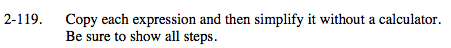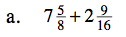This sum can be represented with a diagram:

Find a common denominator to add the two fractions.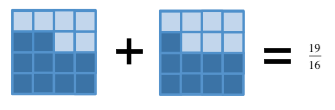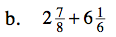See part (a) for strategy.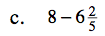Draw 8 squares on your paper like this:

$\text{Cross off }6\frac{2}{5}\text{. What is left? }$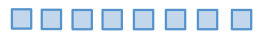$1\frac{3}{5}$